﻿ New Formulas and Mechanism for the Spiral Arm Formation of GalaxiesPublications are Open
Access in this journal
Article Versions
Export Article
• Normal Style
• MLA Style
• APA Style
• Chicago Style
Research Article
Open Access Peer-reviewed

### New Formulas and Mechanism for the Spiral Arm Formation of Galaxies

Hongjun Pan
International Journal of Physics. 2019, 7(3), 73-85. DOI: 10.12691/ijp-7-3-2
Received August 10, 2019; Revised September 26, 2019; Accepted October 23, 2019

### Abstract

A rotating double-side sprinkle emission model is proposed to describe mechanism for the spiral arm formation of galaxies and various types of spiral patterns. The spiral galaxy can be treated as an ideal fluid system with a universal flat rotation curve; a rotating black hole is located at the center of the galaxy with rotating axis perpendicular to the galactic disc. Inside the black hole, the matter is transformed into new type of matter with anti-gravitational property and emitted by the black hole through two opposite directions in the galactic rotating plane similar to the lighthouse beams, it is then converted to non-gravitational matter. The new matter moves in straightly confined route for certain distance, and then it moves freely with its momentum combining the fluid rotation of the galaxy. The new matter will be transformed to hydrogens on its way of motion, increases the local hydrogen density, refuels the local stars and promotes young star formation, the luminosity of the area is temporarily enhanced, brighter than other areas, such enhanced luminosity area is what seen as the galactic bar and spiral arms. New formulas with one parameter and one variable are derived in three different cases. Different categories of theoretical spiral patterns have been produced and various real images of the spiral galaxies have been precisely simulated by the new formulas. This model seems a universal model and can be applied to most (if not all) spiral galaxies. Galaxies with special spiral patterns like Hoag’s object and arms not meeting the end of galactic bars can be naturally explained by this model. This model is the first attempt to think the spiral arm formation with the hydrogen originated from inside of the galactic center to outside. The proposed mechanism of the hydrogen production seems highly speculative, but the result of the simulations is very satisfactory, this indicates that the idea for the hydrogens originated from inside the bar is a promising approach, may lead us to re-think about the property of the black holes and hope to serve as a trigger to promote the future research in this direction.

### 1. Introduction

The spiral galaxies are the most fascinating objects in the sky because of their beautiful spiral arms extending from the centers to the edges, these arms contain swarms of hot, blue stars, clouds of dust and gas and young star dusters. Our universe is full of different types of galaxies classified with Hubble sequence based on the morphology of the galaxies 1, 2. It is estimated that the number of galaxies in the universe may be up to 2 trillion 3. The spiral galaxies together with irregular galaxies make up approximately 60% of galaxies in today’s universe 4. A spiral galaxy has disk-like morphology, all the stars, gases and stellar medium circulate around the center of the galaxy with differential rotation speeds, and the interesting flat rotation curve does not follow the Kepler’s laws with the estimated mass distribution. The mechanism of the formation of the spiral arm is still unclear. In barred galaxies, the spiral arms prefer binding to the end of galactic bars; this really suggests that the bar plays an important role in the formation of the arms. The formation mechanism of spiral galaxies has been extensively studied in modern astronomy and no satisfied theory has been developed yet. A good model must explain most (if not all) regular and irregular spiral patterns, how they are formed and what patterns can be described. The leading theory in explaining the formation of the spiral pattern is the Density Wave Theory (DWT) with later many variations, it was first proposed by Lin and Shu in 1964 5, 6, this hypothesis has been extensively studied and extended to the explanation of Saturn rings 7, 8, 9, 10. The DWT is limited only to the spiral galaxies which have well defined architectural spiral arm patterns such as grand design galaxies, which are only a small portion of the total, the DWT cannot explain the formation of spiral galaxies with spurs and branches. Several other alternatives or theories such as Manifold theory, Magnetohydrogendynamics theory and N-body simulations were proposed to explain the formation of the spiral arms 11, 12, 13, 14, those models can only be used for few subset of the spiral galaxies, not for broad application. For example, the DWT may be suitable for short arm patterns like NGC1300 types, the locally excited waves due to instability will lose its coherence when they extend to other area and disappear soon, the “instability” self implies a great degree of short lived and random nature, may cause destruction to the already formed spiral arms. In reality, it is unlikely that the galaxies with well-defined long arms such as UGC12158, NGC1398 and NGC4622 were formed by DWT. Furthermore, a very common question regarding the DWT is that how the density wave is initiated or seeded? The DWT model does not provide a convinced answer, instead, with many possible ideas such as internal global density wave (internal instability) 15 or (external) tidal effect by galaxy encounters. It is highly desirable to seek a universal model, which can explain the spiral pattern formation of the most spiral galaxies with satisfactory accuracy.

Regular and well-defined spiral patterns may be described by logarithmic spiral formula with constant pitch angle with the radius. Ringermacher and Mead developed a new mathematic formula which can quantitatively characterize the Hubble sequence of spiral galaxies including grand design and barred spirals 16, but does not provide a physical mechanism to explain how such spiral patterns are generated in the nature. In this paper, a new hypothesis is proposed to explain the formation of the spiral galaxies for all (if not exaggerated) types of regular and irregular spiral galaxies based on the rotating double-side sprinkle emission mechanism. However, irregular galaxies caused by merging, collisions and other external impacts are not included.

### 2. The Rotating Double-side Sprinkle Emission Model for the Spiral Arm Formation of Galaxies

This model is inspired by the striking image of NGC 1300 galaxy shown in Figure 1. In this image, the galaxy has two symmetrically well-defined half-circle visible arms and a galactic bar, the arms and the galactic bar are smoothly and naturally connected and formed one whole object, the density of blue and young stars at the two ends of the bar is much higher than other arm sections. Hydrogen gas is the essential material and fuel for star formation and glowing (burning), therefore, the density of hydrogen gas in such areas is much higher. It is well known that the disc of a spiral galaxy rotates around the galactic center, hydrogen gas is spread everywhere in the galaxy, more or less random distribution is more practically expected. In such rotation system, how can the hydrogen gas is concentrated along the specially preferred symmetric lines (arms) in the galaxy? If we think this phenomenon in opposite way, i.e., the seed for growing the spiral arms is inside of the bar, the extra hydrogen gas in the arms is originated inside of the bar, then, the whole scenario will be totally different and the formation of all (if not exaggerated) types of spiral galaxies can be much more logically explained.

It is highly believed that all spiral galaxies have black holes in their centers, the black holes are very small objects with extremely high density and gravitational force, the matter is swirling into the black holes, nothing within the horizon range can come out of the black holes. So far, we know little about the black holes and it is full of mysteries. However, Hawking proposed that radiation (Hawking radiation) is predicted by black holes due to quantum effects near the event horizon 17, such radiation reduces the mass and rotation energy of black holes, therefore, the black holes will shrink and ultimately vanish if they do not gain mass through other means. Pan proposed that the rotation of the galaxies can be treated as ideal fluid system and followed by both fluid mechanism and gravitational mechanism 18, the gravitational force serves as fluid viscosity and binding force; it is further concluded in that paper that the combination of the fluid mechanics and gravitational mechanics is the only mechanism to restore the galaxies into flat disc shapes with flat rotation curves after galaxy collision or merging. By adapting this ideal fluid concept and evaluating various other aspects, the following rotating double-side sprinkle emission model is proposed for the spiral arm formation of galaxies:

I. A rotating black hole is located in the center of the galaxy with rotating axis perpendicular to the galactic plane, absorbs matter from its surrounding, at the same time, the matter inside of the black hole is transformed to different kind of matter by unknown mechanism due to the extreme physical condition, such different kind of matter may have anti gravitational property and is emitted (expelled) by the black hole through two opposite directions (like south and north poles) in the galactic rotating plane like lighthouse beams as illustrated in Figure 2. The new anti-gravitational matter can be called “Imaginary Matter” (IM) now and can be changed to a more proper name later; it is not the previously proposed dark matter or dark energy. A good analogy to this emission is the pulsar radiation. After the imaginary matter is out of the black hole, it may be transformed to non-gravitational matter and moves straightly for certain distance in a confined route (analogy to magnetic confinement?), then, the imaginary matter move freely with its momentum, the rotating fluid current of the galaxy will carrier the IM flowing with it also, so the final motion of the IM is the combination of the straight outward motion and the galactic rotation. The length of the confined route is the observed half bar length and it is definitely associated with the black hole. This description is very similar to water lines from a rotating double-side garden sprinkle; therefore, this new model for spiral arm formation of the galaxies is named as Rotating Double-Side Sprinkle Emission model, for short, RDSSE model.

II. The IM will be gradually converted to hydrogen atoms during its motion. According to the Big Bang theory, the universe was created by a Big Bang, huge amount of matter was released, after sometime later, hydrogen atoms were generated from such released matter, then, stars, galaxies…. were formed. The process of conversion of the IM into hydrogens could be a mini version of such mechanism. Such matter transformation starts after the IM leaves the black hole beyond the horizon.

III. Due to the fact that hydrogen gas is gravitational material, the newly generated hydrogen gas will be locked by strong gravitational force of the galaxy and cannot move outwards with the IM, it will be carried by the rotating current of the galaxy and rotate around the center of the galaxy. The newly generated hydrogen gas significantly and temporarily increases the hydrogen density in its location (includes the confined route) and refuels the stars in such location and promotes new star formation, therefore, luminosity in those areas is temporarily much higher than other areas. The IM continues moving outwards, the luminosity will decrease and be back to normal condition when the excess hydrogens are depleted after the IM stream moves out the area. The amount of produced hydrogen gas from the IM depends on the amount of IM in the area which will gradually decrease on its way.

• Figure 1. The image of NGC 1300 galaxy
• Figure 2. Illustration of matter conversion and emission by the black hole

### 3. Differential Equations and Solutions for Spiral Arms Formation of the Galaxies

Based on the RDSSE model outlined above, the trajectory of the IM movement can be illustrated in Figure 3 and Figure 4. Assume that the galaxy is an ideal fluid rotation system and follows universal flat rotation curve.

• Figure 3. IM moves through the confined route
• Figure 4. IM moves in the flat rotation region

The green area is the bar rotation area where the rotation velocity linearly increases with radius, and the light blue area is the flat rotation area, where the rotation velocity is constant. The galactic bar is shown by orange area and IM stream move through the bar.

Based on this model, the hydrogens are generated in the IM moving path (yellow path), so when IM moves in the confined route, hydrogens are generated, the stars in the IM path and close to this area get refueled and new stars are formed, the density of stars in such central area is much higher than other galactic area so luminosity is much higher, this is what we see as very bright galactic bar (orange area). At the time t(α) shown in Figure 3, the axis of the confined route (galactic bar) is at the angle α with respect to the Y axis, the emission velocity Ve(α) is the velocity of the IM moving out the confined route (the end of the bar), the Ve(α) is perpendicular to the rotation circle at the point. Vr(α) is the rotation velocity of the galaxy at the same point, Vr(α) is tangent to the rotation circle. The final motion velocity V of the IM is the combination of Ve(α) and Vr(α), the components of V in X direction and in Y direction are: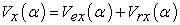(1)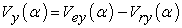(2)

Where, Vex(α) and Vey(α) are the X and Y components of emission velocity Ve(α), and Vrx(α) and Vry(α) are the X and Y components of rotation velocity Vr(α):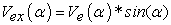(3)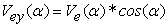(4)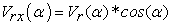(5)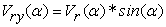(6)

As assumed that the Ve keeps its initial direction and velocity and does not change with time and location for not long distance traveling, so Vex(α) and Vey(α) do not change with time and location, can be just simply written as Vex and Vey. The rotation velocity changes its direction, does not change its value. For a small movement of the IM along the white dash line with velocity V and small time dt period, the new position shown in Figure 4 is at β angle with respect to Y axis,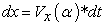(7)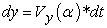(8)

Or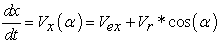(9)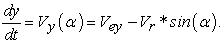(10)

Based on the polar coordinate system,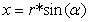(11)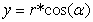(12)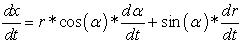(13)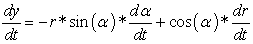(14)

Where, the r is the distance to the center of the galaxy. Equation (9) must equal equation (13), and equation (10) must equal equation (14), two differential equations are derived with proper rearrangement: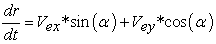(15)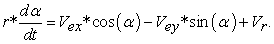(16)

A new differential equation can be derived by dividing equation (15) by equation (16):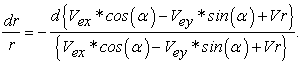(17)

Equation (17) has a solution: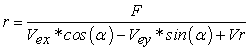(18)

Where, the F is a constant. If the initial position of the galactic bar is aligned with Y axis, α = 0, and r = the half length of the bar Rb , Vex =0, Vey = Ve.

So the F is: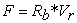(19)

A new parameter ρ is defined as the ratio of the emission velocity over the rotation velocity: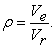(20)

Then,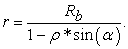(21)

With equation (21), equation (15) can be rewritten as: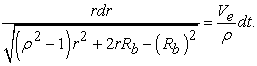(22)

The differential equation (22) can be solved in three different cases, respectively: ρ >1, ρ = 1, and ρ < 1.

Case 1: ρ >1, the solution for the equation (22) is: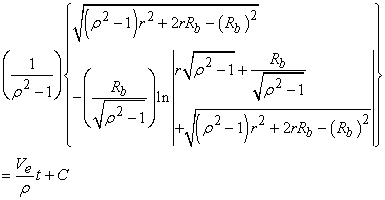(23)

With constant C (when t =0, r = Rb):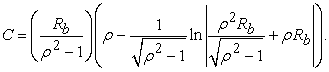(24)

Case 2: ρ = 1, the solution for the equation (22) is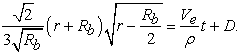(25)

With constant D (when t =0, r = Rb):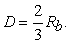(26)

Case 3: ρ < 1, the solution for the equation (22) is: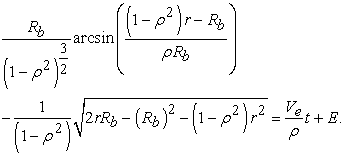(27)

With constant E (when t =0, r = Rb):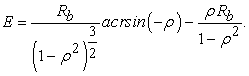(28)

The value of arcsin is in unit of radius not in degree. The angular rotation speed ω of the galactic bar is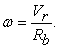(29)

After time t, the bar rotates to the position with the angle θ respect to Y axis,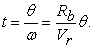(30)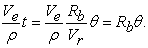(31)

So the rotation angle of the galactic bar is equivalent to the time scale. Put equation (31) into equation (23), equation (25) and equation (27), the final formulas are obtained:

For ρ > 1: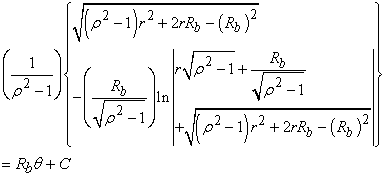(32)

For ρ = 1: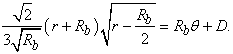(33)

For ρ <1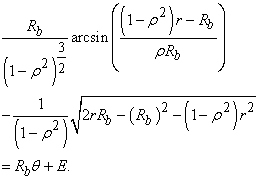(34)

The equations (21), (32), (33) and (34) can be used to calculate different spiral patterns of the galaxies. One can see that the final spiral arm formation equations have only one parameter ρ and one variable r, different ρ will produce different spiral patterns (will be seen in the following sections).

All the equations are derived with assumption that the initial position of the galactic bar is aligned with Y axis, but the IM is continuously emitted during the bar rotation; however, the motion behavior of the IM emitted in other angles is exactly same as the IM emitted with bar aligned with Y axis, the only difference among those different emitted IM is their relative bar rotation angles. The steps to calculate the spiral arm pattern is outlined here:

1. Select the right equation to calculate the rotation angle θ of the bar according to the value of ρ. For example, if ρ > 1, select equation (32) to calculate the θ with variable r. r = Rb represents the current time. If ρ changes with time (rotation angle θ represents time), then use θ as variable to find the matched r.

2. Use equation (21) to calculate the X and Y coordinates of the IM location: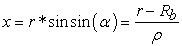(35)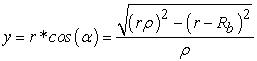(36)

3. Rotate x and y coordinates backward for the rotation angle θ: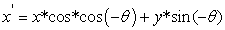(37)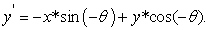(38)

4. Make Euler angle rotation to match the orientation of the galaxies respect to the line of sight.

5. Plot the calculated spiral arm pattern and compare it with real images of galaxies.

### 4. Calculation of Spiral Arm Patterns with Different Categories

The emission velocity Ve and the rotation velocity Vr of the galaxy can change with time. If the Vr changes, the galaxy is not stable, and the spiral pattern is not predictable, so the description of the spiral patterns in this paper will focus on the stable galaxies with flat rotation curves, i.e., the rotation velocity is constant (shown as light blue area in Figure 3 and Figure 4 when the radius beyond the bar rotation radius R).

In the calculation, the half length of the bar is set to 1 arbitrary unit length, and the parameter ρ is the only changeable parameter in the calculation, which can be a constant or changes continuously with time linearly or other formats.

Figure 5 lists 9 different spiral patterns by the calculation with different parameter ρ. Figure 5a is produced with parameter ρ =0.6, it is the spiral-plus-ring pattern classified as SB(r)a. Figure 5b is produced with ρ = 0.7, it is classified as Sa, however, it will be a spiral-plus-ring pattern similar to Figure 5a when the length of arms continues growing. Figure 5c is produced with ρ = 1.4, and is classified as Sb; one can see that the spiral winding gets looser with increase of ρ. Figure 5d is produced with ρ linear increase with backward time with increase rate of 0.0048/degree and initial ρ0 = 1, it is classified as Sc. It has to be clarified that the calculation is from center to edge, this means that when the ρ increases with the backward time, a larger ρ is used in calculation for outer position, a larger ρ for the past means the IM emission velocity is higher in the past with assumption that the rotation of the galaxy is stable. The time evolution sequence is in opposite order, the arm at the end of the galactic bar is current time and the arm at the edge was produced at the end of galactic bar in the past and traveled to that position. Figure 5e is produced with ρ linear increase with backward time with increase rate of 0.05/degree and initial ρ0 = 0.5. One can see that the larger increase rate makes the spiral arms more open. Figure 5f is produced with ρ = 0.56, which will be spiral-plus-ring pattern if the arms get longer. Figure 5g is produced with ρ linear increase with backward time with increase rate of 0.022/degree and initial ρ0 = 1. Figure 5h and Figure 5i is produced with ρ more dramatic increase with backward time by tangent functions. Figure 5b to Figure 5i are produced for comparison with Ringermacher and Mead’s result (which is not reproduced here for respecting to the copy right, readers shall check the reference for comparison) (Ringermacher and Mead 2009), the results by two different methods match each other very well.

The density of the stars and dust clouds in the spiral galaxies gradually decreases with distance to the galactic center; the density of IM gradually decreases when it moves outward with conversion to hydrogen gas and possible to other materials, the density of IM even reduces further due to spreading and diffusing, therefore, less and less hydrogen is produced, the luminosity of the arm gradually decreases and fades away when the arms moves outwards. When the distance is large enough (larger than the visible limit radius L), the sections of arms are invisible as indicated in Figure 6. In reality, less than 2 winding spiral arms are commonly observed. The spiral arms and the galactic bar together (more precisely, the higher luminosity band) will rotate as a “rigid” pattern, the rotation of the stars and dust clouds in the spiral arms is not affected by the spiral arms.

• Figure 5. 9 different spiral patterns produced by the model. The parameters for generating each pattern are listed below: a: ρ = 0.6; b: ρ = 0.7; c: ρ = 1.4; d: ρ = 1*(1+0.0048*θ); e: ρ = 0.5*(1+0.05*θ); f: ρ = 0.56; g: ρ = 1*(1 + 0.022*θ); h: ρ = 1*tg(0.17*θ); i: ρ = 0.6 *tg(0.28*θ)
• Figure 6. Faded and invisible spiral arms
• Figure 7. A galaxy without spiral arms
• Figure 8. A galaxy with spiral arms

Figure 7 and 8 illustrate the galaxies without and with spiral arms, respectively, the spiral arm are just higher luminosity band, an optical effect.

The spiral-plus-ring patterns like Figure 5a are very interesting. Refer to the equation (21), the distance r must be positive, the maximum value of sin(α) is 1 and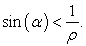For ρ <1, the maximum angle of α is 90 degree and the maximum distance r is: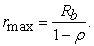(39)

This is the spiral-plus-ring pattern, rmax is the radius of the ring, the ring size increases with the parameter ρ.

For ρ = 1, the ring size is infinitive, i.e, no ring pattern, r has no limit.

For ρ > 1, the following condition must be followed:(40)

Therefore, the angle α of IM respect to its initial emission axis is limited, but the r has no limit.

Figure 9 shows three different spiral-plus-ring patterns with ρ = 0.4, ρ = 0.6 and ρ = 0.8, respectively.

The number of spiral windings increases with parameter ρ; when ρ -> 0, the radius of the ring approaches to Rb, this could be responsible for the inner ring structures in some galaxies such as NGC 1398 and Milky May’s near 3kpc arm and far 3kpc arm if the IM has small portion with very slow emission velocity; when the ρ -> 1, the radius of the spiral ring approaches to infinity. However, in reality, the most (if not all) observed spiral galaxies have less than two windings.

### 5. Simulations for Real Galaxies

The best test for the proposed hypothesis is to check if it can successfully simulate different types of spiral arm patterns of galaxies. The simulations are calculated with arbitrary units of length and time, the rotation angle of the galactic bar is used as time scale, and the graphs are scaled up or down to match the images of galaxies.

5.1. UGC12158

Figure 10 shows the simulation (right) by the RDSSE model compared to the original image (left). The image of the galaxy is face-on image, the simulation fits the spiral arm pattern very well, it is a very regular spiral pattern with parameter ρ = 1, i.e., the IM emission velocity equals to the galactic flat rotation velocity.

• Figure 9. The ring sizes of spiral-plus-ring patterns with different ρ values
• Figure 10. UGC 12158, type: Sb, ρ = 1.0, Euler(38,0,0)
• Figure 11. NGC 2273, Type SB(r)a, left, orginal image and fitting, ρ = 0.6, Euler angle (-43, 57, 80). Right, face-on image (right) converted form the original image and fitting, ρ= 0.6
• Figure 12. NGC 1398, type: (R')SB(r)ab, ρ = 0.55*(1 + 6.8*10-72), Euler(7,48,0)
5.2. NGC2273

Figure 11 shows the simulation by this model with comparison to the original image. The image of this galaxy shows spiral-plus-ring pattern, the left above is the original image and the left below is the simulation with ρ = 0.6 and proper Euler rotation. The right above is the face-on image converted from the original (left) image and the below is the simulation. One can see that the simulation matches the original image very well.

5.3. NGC 1398

Figure 12 shows the simulation (right) for the NGC 1398 compared to its original image (left). The spiral pattern of this galaxy is very beautiful and also called Ribbon and Pearl galaxy. It can be simulated with ρ changing with backward time by equation: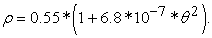(41)

The equation (41) tells us some useful information about the evolution of the galaxy. The current value of ρ is 0.55, the spiral arm pattern should look like Figure 5a, a spiral-plus-ring pattern if ρ was constant through its history to the past; but its ρ gradually increased with the backward time, i.e., the ρ (which reflects the IM emission velocity with assumption that the rotation velocity remains stable) has been gradually decreasing from past to now, the decrease rate depends on the square of the time with very small coefficient 6.8 * 10-7. The inner ring could be formed by hydrogens converted from a portion of the IM which has very slow emission velocity or by other mechanism. The most arms near the edge are broken into pieces like spurs due to many reasons which may include but not limited to: 1. The galaxy is not a perfect ideal fluid system; 2. Local chaos and disturbance in matter distribution and motion velocity; 3. IM spreads and diffuses to the galactic fluid system. The IM band gradually loses its coherence on its moving path under the effect of those reasons; the edge arms will gradually fade and disappear in the future when they move out the visible range. Those reasons may also cause the formation of spurs, branches and feathers of the spiral arms in the galaxies like famous pinwheel galaxy M101. This topic was studied in detail by Chakrabarti et al. 19.

5.4. HOAG’s Object

The Hoag’s Object shown in Figure 13 is a very interesting galaxy, it has a ring pattern with spherical bugle in the center without visible bar. The pattern can be easily explained by the RDSSE model. This galaxy was a spiral-plus-ring pattern like Figure 5a in the past, however, the central black hole stopped emitting IM at some time ago, the spiral portion swirled to the ring and the bar shrank and disappeared due to depletion of hydrogen gas, only central bugle remains. The ring seems about to break into pieces similar to NGC 1398, will disappear by spreading/diffusing and depletion of extra hydrogen gas in the future if no more IM emission occurs.

• Figure 14. NGC 1300, ρ = 0.65, Euler ( -25, 55,0)
5.5. NGC 1300

This galaxy is classified as (R')SB(s)bc. Figure 14 hows the simulation for this galaxy with ρ = 0.65. With this ρ value, it could be a spiral-plus-ring pattern like Figure 5a, but the visible arm length is not long enough in this image.

5.6. M51

M51 is a whirlpool galaxy classified as SA(s)bc. The spiral arms have two very different patterns which reflect that its evolution history has two different periods in such visible pattern image. The whole arms can be simulated with 2 section fittings shown in Figure 15. Its later time arm portion is similar to Figure 5e with very open spiral arms and can be fit by equation: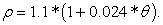(42)

The simulation is shown with solid red lines, the bar rotation angle is about 485 degrees for this period. The earlier time portion can be simulated by the equation: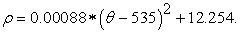(43)

The simulation is shown by the yellow lines, the bar rotation angle for this period is 125 degrees. This portion of the arms is still more or less symmetric respect to the galactic center, therefore, it is the M51 internal change, not by external impact. Obviously, the evolution dynamics in the two sections is significantly different. Morphologically, no bar is apparently observed; however, it may still have a bar in the center with very short length.

• Figure 15. M51 Two section fitting, Euler(13,0,0)

Figure 16 shows the parameter ρ (equivalent to IM emission velocity) change with time, the rotation angle also serves as time scale. This figure tells us that the IM emission velocity in the past gradually decreased, then, at rotation angle 535 degree from current, the IM emission increased for short period of time (about 50 degree rotation time), then, it has been steadily decreasing up to today.

For irregular spiral patterns, the evolution of the galaxies could be different in each section of the pattern, therefore, multiple section simulation can be used to simulate the spiral patterns and useful information about the history could be extracted.

• Figure 16. Parameter ρ changes with time for M51 galaxy (Rotation angle of the galactic bar (in degree), (Equivalent to the time scale from the past to current))
5.7. UGC 6093

The image of UGC 6093 clearly shows that the spiral arms has two distinguishable sections, which can be simulated nicely by the proposed model shown in the Figure 17 by solid lines and dash lines, respectively. It clearly shows that the dash portion of spiral pattern of the galaxy is like Figure 5e with more open arms in which the parameter ρ gradually decreased with forward time for 80-degree rotation time, this section of arms can be simulated by the equation: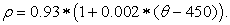(44)

From the rotation angle 450 degree time in the past to the current time, the emission stabilized with constant ρ = 0.93. Due to the spiral arms swirling outwards, the dash sections will move out of the visible range and disappear in the future after another 260 degree rotation time if the parameter ρ remains the same value.

• Figure 17. UGC 6093, two section simulations, Euler (57, 0, 0)
5.8. NGC 4622

NGC 4622 is a face-on unbarred spiral galaxy and classified as SA(r)ab shown in Figure 18. It shows backward winding in the inner area. The two leading spiral arms can be simulated by the equations: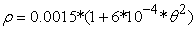(45)

for spiral arm yellow simulation; and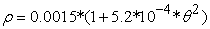(46)

for spiral arm red simulation. The simulation equations indicate that the parameter ρ gradually decrease from past to sometime close to current time with square of the time in both arms, the two opposite IM emissions were not equal in the past, the velocity of the IM emission for yellow simulation arm was slightly higher than IM emission for red simulation arm, but the emission decrease of yellow simulation arm was slightly faster than the red simulation arm. The black hole stopped IM emission at some time in the past, so the galactic bar shrank and disappeared, only central bugle remains.

• Figure 18. NGC 4622, non-equal simulation for both arms
5.9. NGC 4731

This galaxy is classified as E6 shown in Figure 19. The pattern can be well simulated by the following equation: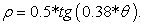(47)
• Figure 19. NGC 4731, Type: E6, ρ = 0.5*tg(0.38*θ), Euler (18, 0, 0)

### 6. Galaxies with Spiral Arms not Meeting the Ends of Galactic Bars

The proposed model predicts that the spiral arms may not always meet the ends of galactic bars when the IM emission changes. As indicated in galaxies Hoag’s object and NGC 4622, the black holes may stop emitting IM, this will cause disconnection of arms from the bars, the bars still rotate and the spiral arms still swirl outwards, rotation gaps between arms and the end of bars occur, such gaps increase with bar rotation. Such scenario can give a false appearance that the arms are ahead of the bars as illustrated in Figure 20 A with green line gaps. The actual scenario is that the arms are behind of the ends of bars shown in Figure 20 B, the blue line gaps are missing sections of the arms due to that the black hole stops emitting IM. The galaxy UGC 3900 may have such scenario 20. If the situation continues, the bar will shrink and disappear in the future.

• Figure 20. A, false appearance of arms ahead of the bar; B: arms behind the bar
• Figure 21. Spiral arm patterns with IM emission velocity decrease with the backward time

### 7. IM emission Velocity Decreases with the Backward Time

Several examples above show that when the IM emission velocity increase with the backward time, the spiral arm pattern will be more open; when the IM emission velocity decreases with the backward time, the spiral arm pattern will be quite different. Figure 21 shows 3 spiral patterns with different IM emission velocities decreasing with the backward time.

Figure 21 A shows a regular spiral pattern with constant ρ = 1. Figure 21 B shows that when the IM emission velocity decrease with the backward time, the spiral arm pattern will be more tightly winding like Figure 21 B, when the decrease rate is larger, the spiral arms will cross each other and spiral arm pattern will be complicated like Figure 21 C, the actual morphology of Figure 21 B and C may be more close to a spiral-plus-ring pattern, such pattern may also cause fake appearance of backward winding. NGC4622 could be suspicious with such scenario. The spiral patterns will be more complicated if the IM emission velocity changes in both increase and decrease directions in the history.

### 8. Discussion

One can see that the rotating double-side sprinkle emission model provides new formulas to simulate all (hope not exaggerated) types of spiral galaxies with a physical mechanism to explain how such patterns are formed. It also naturally explains some unusual galaxy patterns such as the Hoag’s object and galaxies with broken connection between the arms and galactic bars because there is no good explanation for such patterns at current time. This model seems to be a universal model, and can be applied to regular and irregular spiral galaxies. The simulation is mathematically very simple, one parameter and one variable; this reflects that the nature is made of “simple and must be simple. Einstein’s attempt of unified field theory is to find such “simple”. The type of spiral patterns depends on only the behavior of the parameter ρ, and the behavior of the ρ may provide important information about evolution dynamics and history of the galaxies. Please note, during the simulation calculation as outlined above, the x may have to be changed to –x, or x may be changed to y (or –y) and y is changed to x (or –x) in equations (35) and (36), depends on the orientation of the bar and the rotation direction of the real galaxy images. The spiral arms and the galactic bar are the enhanced luminosity caused by temporary density increase of hydrogen gas produced from IM when the IM band passes the area in which stars get refueled and new stars are formed with the hydrogens produced in those area, it is an optical effect, not a real material object; the galactic bars and the spiral arms together rotate as a whole rigid pattern. This model is the first time proposed and is full of imagination, and may have many defects; however, sometimes, imagination is more important than knowledge, it can take people to the place far beyond the limit of knowledge. For sure, many questions may arise regarding this hypothesis, such as “how is the matter converted to anti gravitational matter inside the black hole, then later to the hydrogen gas?”, “what is the confined route”, etc. Those questions are equivalent to the questions “why and how did the Big Bang happen? And how was the hydrogen created after the Big Bang?”, there are no answers at this time, extensive research in the future may provide answers, or may never have answers. The mechanism for the production of the hydrogen in this model seems highly speculative, especially for something coming out of the black hole; but the result of the simulations is very satisfactory, such result may lead us to re-think about the property of the black holes. This is the first attempt to find the seed for the formation of spiral arms inside of the bar, can serve as a trigger to promote future research in such direction and find better seeds. For the density wave theory, it is also a highly speculative model, many assumptions have to be applied during the model development and simulation calculations, it can only be applied to small portion of the spiral galaxies with well-defined patterns. The new formulas derived from the model proposed in this paper can precisely describe spiral patterns as demonstrated above, and any proposed models in the future should match or exceed this accuracy level. It will be appropriate to use Bertrand Russell’s words to finish this paper: The world is full of magical things patiently waiting our wits to grow sharper.

### 9. Conclusion

At least in morphology, the proposed rotating double-side sprinkle emission model successfully described various spiral patterns of galaxies with new formulas; and it also provided a new mechanism to explain how those patterns are formed; however, such mechanism has to be verified. The spiral arms and the galactic bars are just the bands with enhanced luminosity, which is an optical effect; the spiral arms and galactic bar rotate together as a “rigid” pattern, has no effect on the motion of local stars and dust clouds. If the rotation of the galaxy is stable, then the spiral pattern will be decided only by the ratio of the new matter emission velocity over the flat rotation velocity of the galaxy, a small ratio produces spiral-plus-ring patterns; a large ratio produces more loose winding patterns; if the ratio gradually decreases with forward time, the spiral arm patterns will be more open. The spiral patterns produced by this model are compatible to the results of previously proposed mathematic formula. It successfully and precisely simulated several different types of galaxies and some interesting evolution dynamics and history of the galaxies may be obtained. It provides a natural explanation for the Hoag’s object and galaxies with arms not meeting the end of galactic bars. Multi-section simulation can be used to irregular spiral galaxies. This model seems to be a universal model and can be applied to most (if not all) spiral galaxies. This model is at the primary stage, may have many defects; many possible questions may arise regarding the model which may not have answers at this time, further study is needed.

### References

  Hubble, E. P. (1927). "The Classification of Spiral Nebulae". The Observatory. 50: 276. In article  Hubble, E.P. 1936, Mrs. Hepsa Ely Silliman memorial lectures, 25. New Haven: Yale University Press. ISBN 780300025002. In article  Conselice C., Wilkinson A., Duncan K., Mortlock, A.,., “The Evolution of Galaxy Number Density at z < 8 and Its Implications”, 2016, ApJ, 830, 83 In article View Article  Loveday J., "The APM Bright Galaxy Catalogue", 1996, MNRAS, 278, 1025. In article View Article  Lin C., Shu F., "On the spiral structure of disk galaxies". 1964 ApJ. 140, 646 In article View Article  Shu F., "Six Decades of Spiral Density Wave Theory". 2016, Annu. Rev. Astron. Astrophys. 54, 667. In article View Article  Goldreich P., Tremaine S., "The formation of the Cassini division in Saturn's rings". 1978, Icarus. Elsevier Science. 34, 240. In article View Article  Goldreich P., Tremaine S., "The Dynamics of Planetary Rings". 1982, Annu. Rev. Astron. Astrophys. 20, 249 In article View Article  Shu F., "Waves in planetary rings". 1984, Planetary Rings, University of Arizona Press. pp 513 In article  Roberts W.,” Large-Scale Shock Formation in Spiral Galaxies and its Implications on Star Formation” 1969, ApJ., 158, 123. In article View Article  Contopoulos G., Grosbol P., “Stellar dynamics of spiral galaxies: nonlinear effects at the ¼ resonance”. 1986, Astron. Astrophys. 155, 11. In article  Contopoulos G., Grosbol P., “Stellar dynamics of spiral galaxies: self-consistent models”. 1988, Astron. Astrophys. 197, 83. In article  Binney J., Tremaine S., 1987 Galactic Dynamics (Princeton, NJ: Princeton University Press). In article  Sellwood J., Sparke L.,” Pattern speeds in barred spiral galaxies”, 1988, MNRAS, 231, 25 In article View Article  Sellwood J., Carlberg R., “Spiral instabilitys: mechanism for recurrence”, 2019, MNRAS., 489, 116. In article View Article  Ringermacher H., Mead L., “A new formula describing the scaffold structure of spiral galaxies”,2009, MNRAS., 397, 164. In article View Article  Hawking S., “Black hole explosions?” 1974, Nature, 248, 30. In article View Article  Pan, H., “The Flat Rotation Curve of the Spiral Galaxies may be the Result of the Perfect Combination of the Fluid Mechanics and the Gravitational Mechanics”. 2019, IJP, 7(2), 44. In article  Chakrabarti S., Laughlin G., Shu F., “Branch, Spur, and Feather Formation in Spiral Galaxies”, 2003,  ApJ. 596, 220. In article View Article  Seigar M., James P., “The structure of spiral galaxies — II. Near-infrared properties of spiral arms” 1998, MNRAS, 299, 685. In article View ArticleThis work is licensed under a Creative Commons Attribution 4.0 International License. To view a copy of this license, visit http://creativecommons.org/licenses/by/4.0/